Back to Kemp Acoustics HomeNext: Pressure radiation from a Up: The piston approximation Previous: The piston approximation   Contents

## Pressure radiation from a piston terminated in an infinite baffle

Consider a rigid piston in a rigid infinite baffle as shown in figure 3.1. The piston vibrates uniformly with a sinusoidal velocity of amplitude
normal to the baffle.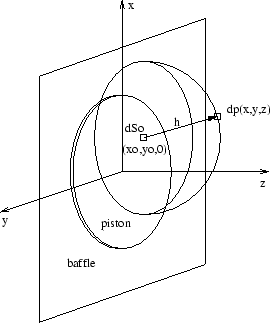In order to calculate the behaviour of this system, we split the piston into infinitesimal simple source elements and sum the resulting pressure fields. A piston surface element of area
is present at. This surface element oscillates with a velocity amplitude ofnormal to the baffle and acts as a simple source of spherical pressure waves. These are represented on the diagram by a hemispherical shell, with the acoustic pressure at a distance
from the source element given by(3.2)

whereis the simple source strength and a
time factor is assumed throughout. The partis known as the Green's function and implies that the pressure oscillates sinusoidally in space with wavelengthand with an amplitude that dies as
. Integrating (3.2) over
, the surface of the whole piston, we get the total pressure field due to the sum of all the source elements that make up the piston.(3.3)

Note that the integrand is singular (tends to infinity) as
tends to zero. This problem must be addressed before numerical integration is possible.

Back to Kemp Acoustics HomeNext: Pressure radiation from a Up: The piston approximation Previous: The piston approximation   Contents
Jonathan Kemp 2003-03-24